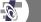PI ETA Consulting Company
 Home Our Singapore Our Lifestyle Food Paradise Shopping Zone Tourist Guide Alpha Explorer Funny BoneManna | ABOUT GEMATRIA More Manna

ABOUT GEMATRIA AND HEBREW CHARACTERS AND THE NUMERIC VALUES

Gematria is the substitution of numbers, or other letters, for letters of the Hebrew alphabet, according to a set system. The purpose is to derive insights into the sacred writings, or to obtain interpretations of the text.

"Ragil" (Normal)

Of the twenty two letters in the Hebrew alphabet, the first nine are numbered consecutively from 1 to 9, the next nine from 10 to 90 in intervals of ten, while the last four letters equal 100, 200, 300 and 400 respectively. This metod is the basis for all other methods. Each letter of the alphabet has its own numerical value. The numerical value of a word or phrase is the sum of the numerical value of all its letter. For example SLWm Shalom equals 376, (S = 300, L = 30, W = 6, M = 40), 300+30+6+40=376.

In "Mispar Gadol" method counts the final form of letters of the alphabet as a continuation of the alphabet (k = 500, m = 600, n = 700, p = 800 and x = 900). In all the other letters have the same value as their normal form (e.g. K = 20, M = 40).

"Millui" (Filling)

Each letter has the numerical value of the sum of the "Ragil" values of all the letters that make up the names of the letter. For example A (ALp Alef ) has the value of 111, because A = 1, L = 30, P = 80, 1+30+80=111.

 A = aleph = 1 / 111 B = beit = 2 / 412 G = gimel = 3 / 83 D = dalet = 4 / 434 H = he = 5 / 6 W = vav = 6 / 12 Z = zayin = 7 / 67 h = chet = 8 / 418 t = tet = 9 / 419 Y = yod = 10 / 20 K = kaf = 20 / 100 L = lamed = 30 / 74 M = mem = 40 / 80 N = nun = 50 / 106 s = samech = 60 / 120 a = 'ayin = 70 / 130 P = pe = 80 / 81 X = tsadi = 90 / 104 Q = kuph = 100 / 186 R = reish = 200 / 510 S = shin = 300 / 360 T = tav = 400 / 406 k = kaph sofit = 500 / 656 m = mem sofit = 600 / 636 n = nun sofit = 700 / 662 p = pe sofit = 800 / 637 x = tsadi sofit = 900 / 660

The words which are calculated using the numeric value of letters is normally written: GYMtRYA. Where as the words which are calculated using the names of the letters ("filling") are written with colons between letters: Q:B:L:H. Final letters are enlarged while they are considered: AMn or A:M:n.

Psalm 34: 2-22: